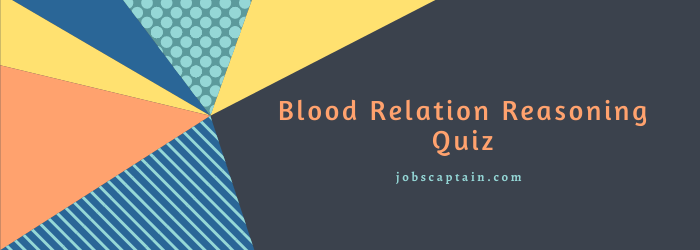# Family Relationship Riddle Reasoning 6This Quiz contain the Reasoning of Blood Relations Riddle questions with answer.  In the Relationships Riddle, relations between certain members of a family are described. Based on this the candidate is expected to answer the given questions.

The main difference in questions of Mixed-Up Blood Relation Descriptions and Relation Riddle type is that in the former, the relationship are described in a round, about and complicated manner.

For Example like ‘the son of my father’s only son’, “His mother is the only daughter of your father.” etc. Where as in the later the descriptions are simple but the number of Blood Relationships are more.

A solved example of the Blood Relationships Riddle is given below for understanding the concept.

Question :  A’s father Y is R’s paternal uncle and S’s husband Z is A’s paternal uncle. How is S related to R?

(A) Cousin

(B) Mother

(C) Sister

(D) Mother

(B) Mother

Y is the paternal uncle of R means Y is the brother of A’s father. So, R’s father and A’s father are brothers.

Also, Z is the paternal uncle of A means Z is the brother of A’s father. So, Z is the father of R. Since S is the wife of Z, so S is the mother of R. Hence, the answer is (B) Mother.

Question : Kanta is mother-in-law of Rita who is sister-in-law of Vinay. Dashahrat is father of Sham, the only brother of Vinay. How is Kanta related to Sham?

(A) Mother

(B) Wife

(C) Mother-in-law

(D) Aunt

(A) Mother

Rita is sister-in-law of Vinay means Vinay is the brother of Rita’s husband. But Vinay has only one brother, Sham. So, Sham is Rita’s husband.

Kanta is mother-in-law of Rita means Kanta is the mother of Rita’s husband i.e. Sham. So Kanta is the mother of Sham. Hence, the answer is (A) Mother.

Question : In a joint family, there are father, mother, 3 married daughters and one bachelor son. Of the daughters, two have 2 sons each, and one has a daughter. How many male members are there in the family?

(A) 3

(B) 2

(C) 6

(D) 9

(D) 9

The male members in the family are father, husbands of 3 married daughters, unmarried son and 2 sons of each of two daughters. So number of male members = (1+ 3 + 1 + 2 x 2) = 9. Hence, answer is (D) 9.

Question : J is the son-in-law of G and also the brother-in-law of K who is the brother of M. How K is related to G?

(A) Uncle

(B) Brother

(C) Father

(D) Son

(D) Son

J is the son-in-law of G and also brother-in-law of K. It means that G is the father /mother of J’s wife and K is the brother of J’s wife. Thus K is son of G. Hence, answer is (D) Son.

Question :

Statement : A is the son of P. F is the P’s sister. F has a son T and a daughter C. R is the maternal uncle of T.

(i) How is A related to T?

(A) Cousin Brother

(B) Nephew

(C) Brother

(D) Uncle

(A) Cousin Brother

A is the cousin brother of T. Hence, answer is (A) Cousin Brother.

Statement : A is the son of P. F is the P’s sister. F has a son T and a daughter C. R is the maternal uncle of T.

(ii) How is C related to R?

(A) Daughter

(B) Nephew

(C) Niece

(D) Sister

(C) Niece

C is the niece of R. Hence, answer is (C) Niece.

Statement : A is the son of P. F is the P’s sister. F has a son T and a daughter C. R is the maternal uncle of T.

(iii) What it is the relationship of P with C and T?

(A) Paternal Uncle

(B) Father

(C) Brother

(D) Maternal Uncle

(D) Maternal Uncle

P is the maternal uncle of C and T both. Hence, answer is (D) Maternal Uncle.

Statement : A is the son of P. F is the P’s sister. F has a son T and a daughter C. R is the maternal uncle of T.

(iv) How many nephews does R have?

(A) 2

(B) 1

(C) 3

(D) 5

(A) 2

R has two nephews, A and T. Hence, answer is (A) 2.

Statement : A is the son of P. F is the P’s sister. F has a son T and a daughter C. R is the maternal uncle of T.

(v) How many brothers does F have?

(A) 4

(B) 1

(C) 2

(D) 3